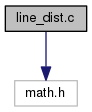GRASS Programmer's Manual  6.5.svn(2014)-r66266
vector/diglib/line_dist.c File Reference
`#include <math.h>`
Include dependency graph for vector/diglib/line_dist.c:Go to the source code of this file.

## Macros

#define ZERO(x)   ((x) < tolerance && (x) > -tolerance)

#define TOLERANCE   1.0e-10

## Functions

int dig_set_distance_to_line_tolerance (double t)

double dig_distance2_point_to_line (double x, double y, double z, double x1, double y1, double z1, double x2, double y2, double z2, int with_z, double *px, double *py, double *pz, double *pdist, int *status)

## Macro Definition Documentation

 #define TOLERANCE   1.0e-10

Definition at line 21 of file vector/diglib/line_dist.c.

Referenced by dig_set_distance_to_line_tolerance().

 #define ZERO ( x ) ((x) < tolerance && (x) > -tolerance)

Definition at line 20 of file vector/diglib/line_dist.c.

Referenced by dig_distance2_point_to_line().

## Function Documentation

 double dig_distance2_point_to_line ( double x, double y, double z, double x1, double y1, double z1, double x2, double y2, double z2, int with_z, double * px, double * py, double * pz, double * pdist, int * status )

Definition at line 45 of file vector/diglib/line_dist.c.

References y, and ZERO.

Referenced by Vect_line_distance(), Vect_snap_line(), and Vect_snap_lines_list().

 int dig_set_distance_to_line_tolerance ( double t )

Definition at line 24 of file vector/diglib/line_dist.c.

References TOLERANCE.